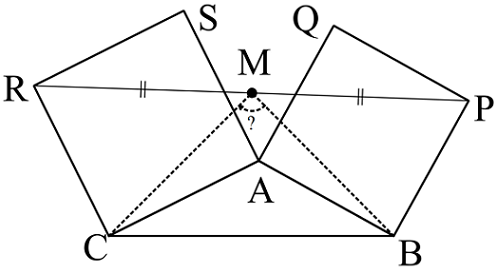# Triangle With Squares!

Geometry Level 4Given a triangle $ABC$, construct two squares $ABPQ$ and $ACRS$. Suppose that $M$ is the midpoint of $PR$. Join $MB$ and $MC$. Find $\angle BMC$.

Details and Assumptions:

• $P$ and $C$ are on opposite side of $AB$.

• $R$ and $B$ are on opposite side of $AC$.

×